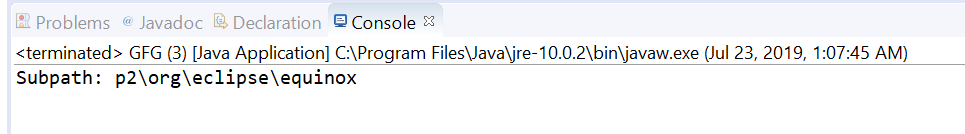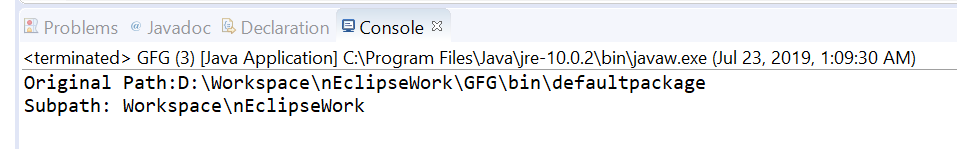# Path subpath() method in Java with Examples

The subpath(int beginIndex, int endIndex) method of java.nio.file.Path used to return a relative Path that is a subsequence of the name elements of this path. We will pass begin and end indexes to construct a subpath. The beginIndex and endIndex parameters specify the subsequence of name elements. The name element closest to the root in the directory hierarchy is index 0 and name element that is farthest from the root has index count-1. The returned subpath object has the name elements that begin at beginIndex and extend to the element at index endIndex-1.

Syntax:

```Path subpath(int beginIndex,
int endIndex)
```

Parameters: This method accepts a two parameters:

• beginIndex which is the index of the first element, inclusive and
• endIndex which is the the index of the last element, exclusive.

Return value: This method returns a new Path object that is a subsequence of the name elements in this Path.

Exception: This method throws IllegalArgumentException if beginIndex is negative, or greater than or equal to the number of elements. If endIndex is less than or equal to beginIndex, or larger than the number of elements.

Below programs illustrate subpath() method:
Program 1:

 `// Java program to demonstrate ` `// java.nio.file.Path.subpath() method ` ` `  `import` `java.nio.file.Path; ` `import` `java.nio.file.Paths; ` `public` `class` `GFG { ` `    ``public` `static` `void` `main(String[] args) ` `    ``{ ` ` `  `        ``// create an object of Path ` `        ``Path path ` `            ``= Paths.get(``"D:\\eclipse\\p2"` `                        ``+ ``"\\org\\eclipse\\equinox\\p2\\core"` `                        ``+ ``"\\cache\\binary"``); ` ` `  `        ``// call subPath() to create a subPath which ` `        ``// begin at index 1 and ends at index 5 ` `        ``Path subPath = path.subpath(``1``, ``5``); ` ` `  `        ``// print result ` `        ``System.out.println(``"Subpath: "` `                           ``+ subPath); ` `    ``} ` `} `

Output:Program 2:

 `// Java program to demonstrate ` `// java.nio.file.Path.subpath() method ` ` `  `import` `java.nio.file.Path; ` `import` `java.nio.file.Paths; ` `public` `class` `GFG { ` `    ``public` `static` `void` `main(String[] args) ` `    ``{ ` ` `  `        ``// create an object of Path ` `        ``Path path ` `            ``= Paths.get(``"D:\\Workspace"` `                        ``+ ``"\\nEclipseWork"` `                        ``+ ``"\\GFG\\bin\\defaultpackage"``); ` ` `  `        ``System.out.println(``"Original Path:"` `                           ``+ path); ` ` `  `        ``// call subPath() to create a subPath which ` `        ``// begin at index 0 and ends at index 2 ` `        ``Path subPath = path.subpath(``0``, ``2``); ` ` `  `        ``// print result ` `        ``System.out.println(``"Subpath: "` `                           ``+ subPath); ` `    ``} ` `} `

Output:My Personal Notes arrow_drop_upI am a Developer I love to code and bring my ideas alive

If you like GeeksforGeeks and would like to contribute, you can also write an article using contribute.geeksforgeeks.org or mail your article to contribute@geeksforgeeks.org. See your article appearing on the GeeksforGeeks main page and help other Geeks.

Please Improve this article if you find anything incorrect by clicking on the "Improve Article" button below.

Article Tags :
Practice Tags :

Be the First to upvote.

Please write to us at contribute@geeksforgeeks.org to report any issue with the above content.